# NCERT Solutions for Class 9 Science Chapter 4 - Structure of the Atom - eSaral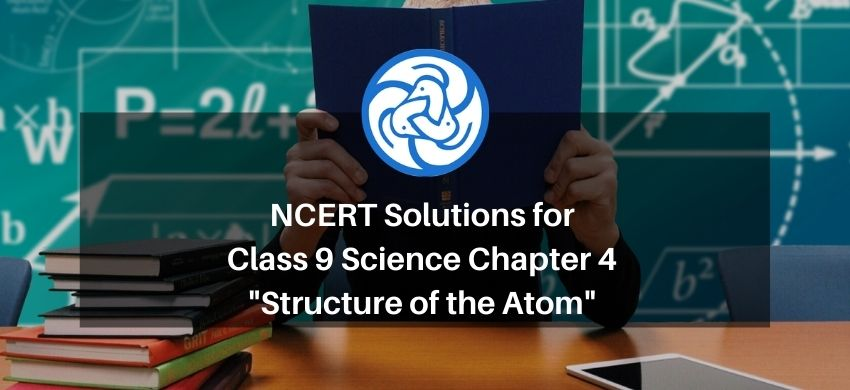Hey, are you a class 9 student and looking for ways to download NCERT Solutions for Class 9 Science Chapter 4 "Structure of the Atom"? If yes. Then read this post till the end.

In this article, we have listed NCERT solutions for class 9 Science Chemistry Chapter 4 in PDF that is prepared by Kota’s top expert Chemistry Faculties by keeping Simplicity in mind.

If you want to learn and understand class 9 Science chapter 4 "Structure of the Atom" in an easy way then you can use these solutions PDF.

NCERT Solutions helps students to Practice important concepts of subjects easily. Class 9 Science solutions provide detailed explanations of all the NCERT exercise questions that students can use to clear their doubts instantly.

If you want to score high in your class 9 Science Exam then it is very important for you to have a good knowledge of all the important topics, so to learn and practice those topics you can use eSaral NCERT Solutions.

So, without wasting more time Let’s start.

### Download The PDF of NCERT Solutions for Class 9 Science Chapter 4 "Structure of the Atom"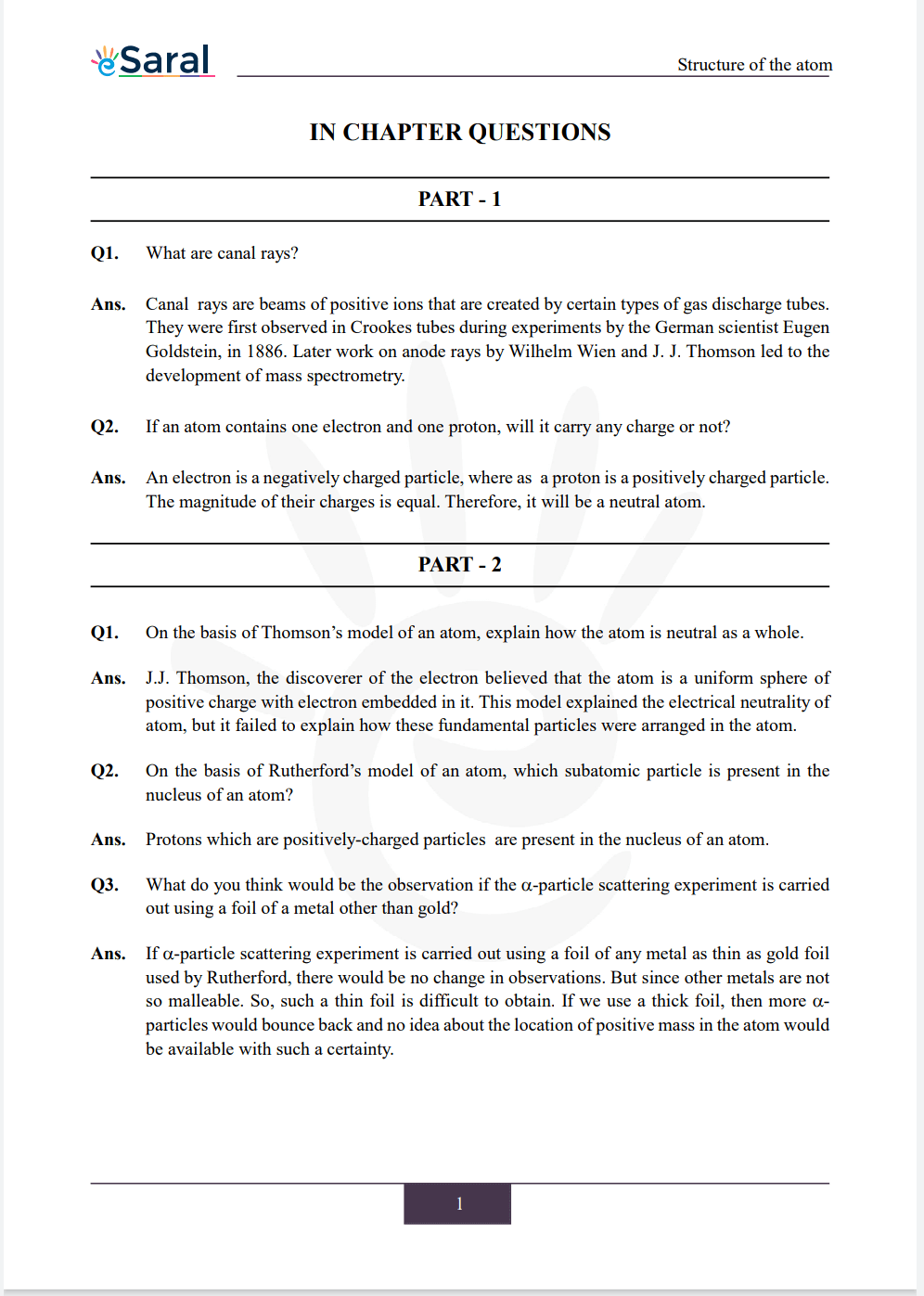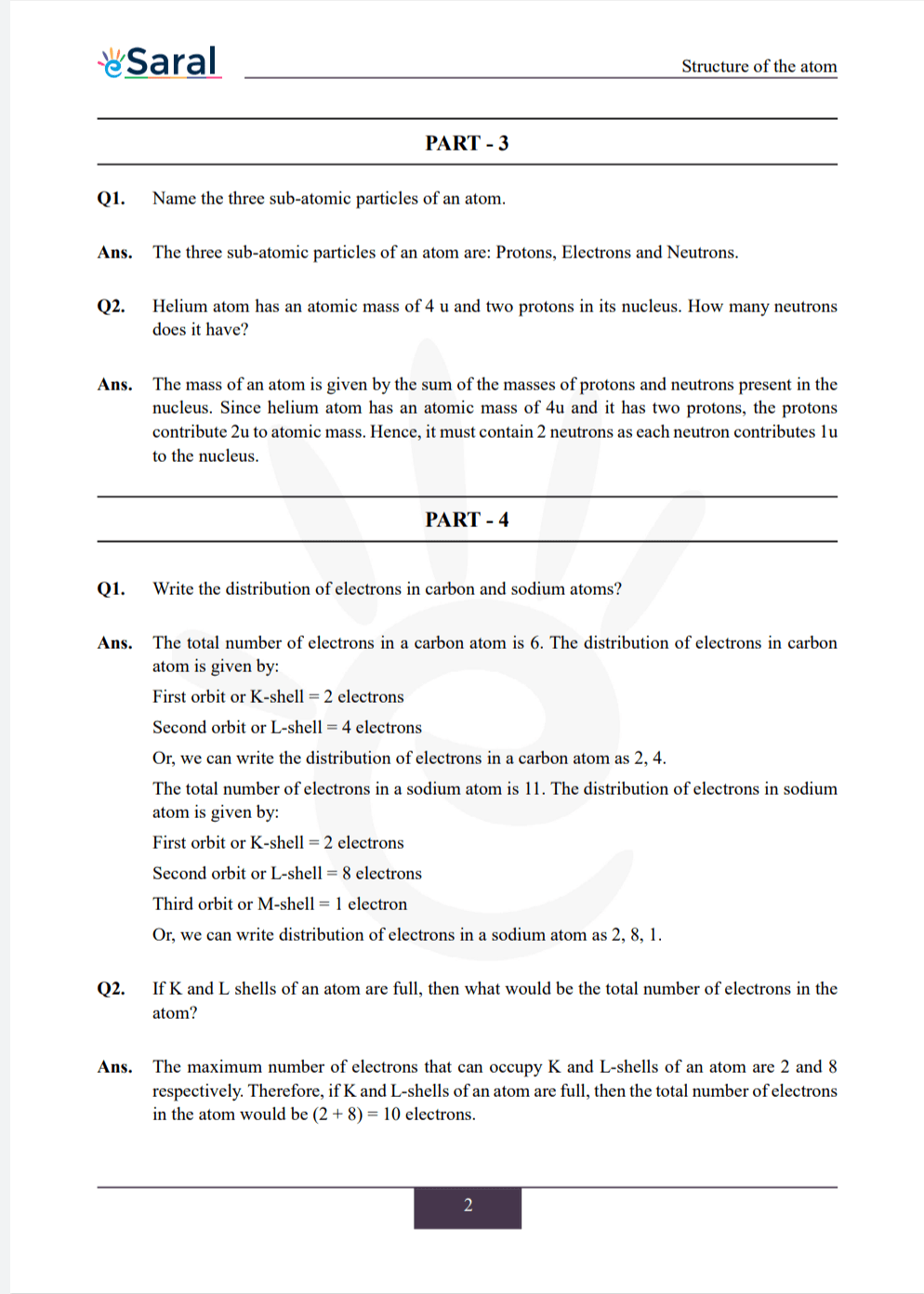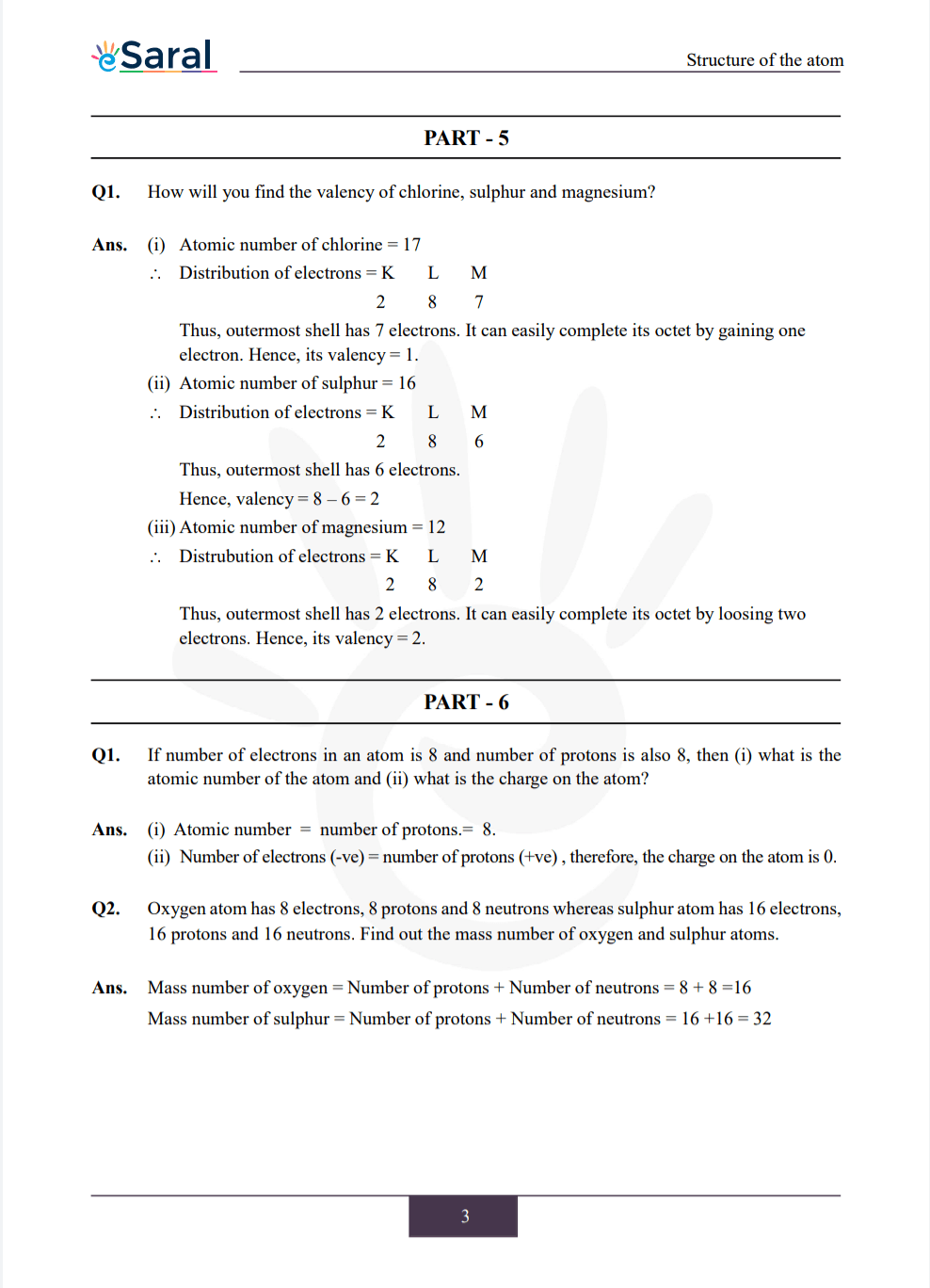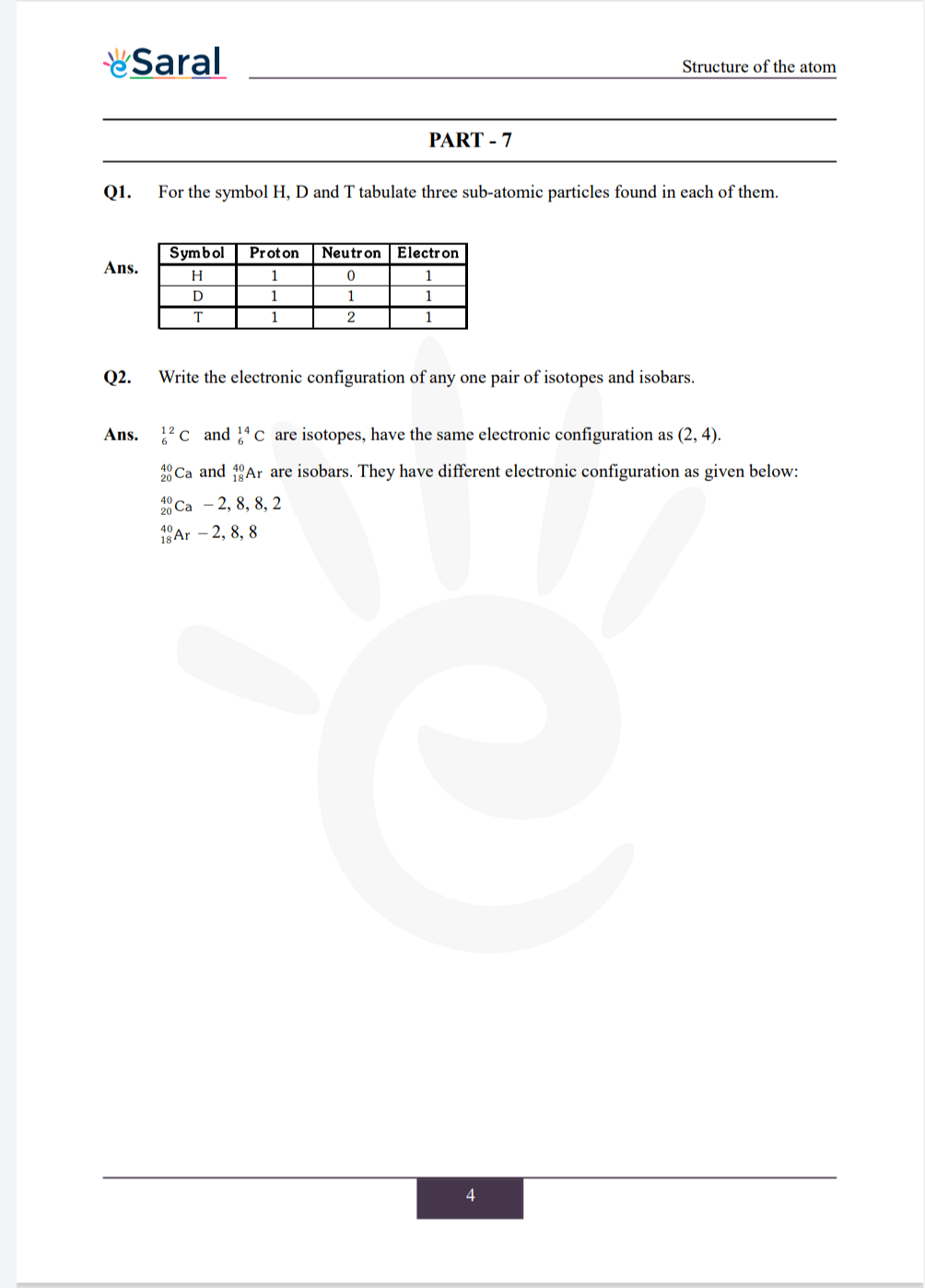### Important topics of class 9 Science Chapter 4

• Structure of Atom
• Valency
• Charged Particles in Matter
• How are Electrons Distributed in Different Orbits
• Atomic Number and Mass Number

#### All Questions of Chapter 4

Once you complete the chapter 4 then you can revise it by solving following questions.

Q1. What are canal rays?

Q2. If an atom contains one electron and one proton, will it carry any charge or not?

##### Part 2

Q1. On the basis of Thomson’s model of an atom, explain how the atom is neutral as a whole.

Q2. On the basis of Rutherford’s model of an atom, which subatomic particle is present in the nucleus of an atom?

Q3. What do you think would be the observation if the -particle scattering experiment is carried out using a foil of a metal other than gold?

##### Part 3

Q1. Name the three sub-atomic particles of an atom.

Q2. Helium atom has an atomic mass of 4 u and two protons in its nucleus. How many neutrons does it have?

##### Part 4

Q1. Write the distribution of electrons in carbon and sodium atoms?

Q2. If K and L shells of an atom are full, then what would be the total number of electrons in the atom?

##### Part 5

Q1. How will you find the valency of chlorine, Sulphur and magnesium?

##### Part 6

Q1. If number of electrons in an atom is 8 and number of protons is also 8, then (i) what is the atomic number of the atom and (ii) what is the charge on the atom?

Q2. Oxygen atom has 8 electrons, 8 protons and 8 neutrons whereas sulphur atom has 16 electrons, 16 protons and 16 neutrons. Find out the mass number of oxygen and sulphur atoms.

##### Part 7

Q1. For the symbol H, D and T tabulate three sub-atomic particles found in each of them.

Q2. Write the electronic configuration of any one pair of isotopes and isobars.

##### Exercise

Q1. Compare the properties of electrons, protons and neutrons.

Q2. What are the limitations of J.J. Thomson’s model of the atom?

Q3. What are the limitations of Rutherford’s model of the atom?

Q4. Describe Bohr’s model of the atom.

Q5. Compare all the proposed models of an atom given in this chapter.
Thomson’s model, Rutherford’s model, and Bohr’s model

Q6. Summarize the rules for writing of distribution of electrons in various shells for the first eighteen elements.

Q7. Define valency by taking examples of silicon and oxygen.

Q8. Explain with examples (i) Atomic number, (ii) Mass number, (iii) Isotopes and (iv) Isobars. Give any two uses of isotopes.

Q9. $\mathrm{Na}^{+}$ has completely filled $\mathrm{K}$ and $\mathrm{L}$ shells. Explain.

Q10. If bromine atom is available in the form of $\mathrm{Br}^{79}, \mathrm{Br}^{81}$, say, two isotopes $(49.7 \%)$ and $(50.3 \%)$, calculate the average atomic mass of bromine atom.

Q11. The average atomic mass of a sample of an element $X$ is $16.2 \mathrm{u}$. What are the percentages of isotopes ${ }_{8}^{16} \mathrm{X}$ and ${ }_{\mathrm{g}}^{18} \mathrm{X}$ in the sample?

Q12. If $Z=3$, what would be the valency of the element? Also, name the element.

Q13. Composition of the nuclei of two atomic species X and Y are given as under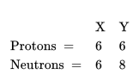Give the mass numbers of X and Y. What is the relation between the two species?

Q14. For the following statements, write $\mathrm{T}$ for 'True' and $\mathrm{F}$ for 'False'.
(a) J.J. Thomson proposed that the nucleus of an atom contains only nucleons.
(b) A neutron is formed by an electron and a proton combining together. Therefore, it is neutral.
(c) The mass of an electron is about $\frac{1}{2000}$ times that of proton.
(d) An isotope of iodine is used for making tincture iodine, which is used as a medicine.
Q15. Choose the correct answer :
Rutherford’s alpha-particle scattering experiment was responsible for the discovery of
(a) Atomic nucleus (b) Electron (c) Proton (d) Neutron

Q16. Choose the correct answer : Isotopes of an element have
(a) The same physical properties
(b) Different chemical properties
(c) Different number of neutrons
(d) Different atomic numbers

Q17. Choose the correct answer :
Number of valence electrons in $\mathrm{Cl}^{-}$ ion are
(a) 16 (b) 8 (c) 17 (d) 18

Q18. Which one of the following is a correct electronic configuration of sodium?
(a) 2, 8
(b) 8, 2, 1
(c) 2, 1, 8
(d) 2, 8, 1

Q19. Complete the following table.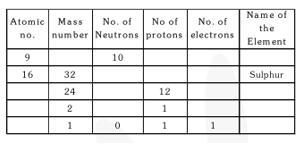Class 9 Science Complete Revision Notes.